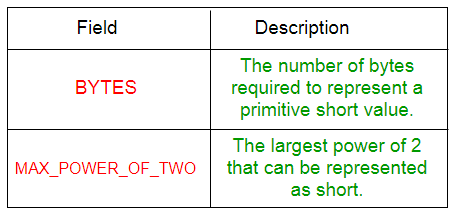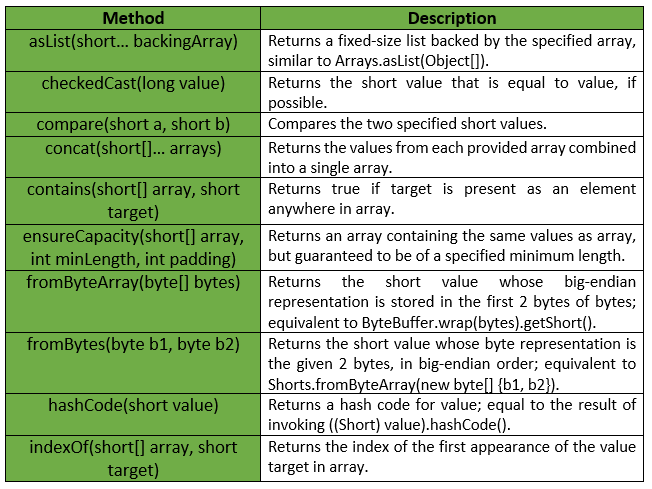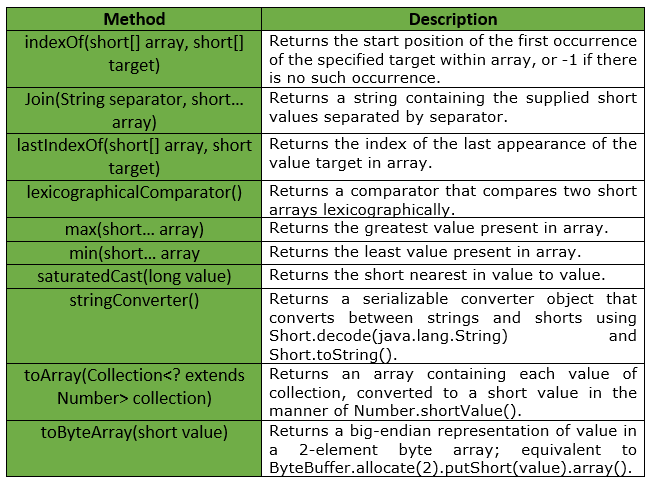Open In App

# Shorts Class | Guava | Java

Shorts is a utility class for primitive type short. It provides Static utility methods pertaining to short primitives, that are not already found in either Short or Arrays.

Declaration :

```@GwtCompatible(emulated=true)
public final class Shorts
extends Object
```

Below table shows the Field summary for Guava Shorts Class :Some of the methods provided by Guava Shorts Class are :Exceptions :

• checkedCast : IllegalArgumentException if value is greater than Short.MAX_VALUE or less than Short.MIN_VALUE
• min : IllegalArgumentException if array is empty.
• max : IllegalArgumentException if array is empty.
• fromByteArray : IllegalArgumentException if bytes has fewer than 2 elements.
• ensureCapacity : IllegalArgumentException if minLength or padding is negative.
• toArray : NullPointerException if collection or any of its elements is null.

Below table shows some other methods provided by Guava Shorts Class :Below given are some examples showing the implementation of Guava Shorts Class methods :
Example 1 :

 `// Java code to show implementation``// of Guava Shorts.asList() method`` ` `import` `com.google.common.primitives.Shorts;``import` `java.util.*;`` ` `class` `GFG {``    ``// Driver method``    ``public` `static` `void` `main(String[] args)``    ``{``        ``short` `arr[] = { ``3``, ``4``, ``5``, ``6``, ``7` `};`` ` `        ``// Using Shorts.asList() method which convert``        ``// array of primitives to array of objects``        ``List myList = Shorts.asList(arr);`` ` `        ``// Displaying the elements``        ``System.out.println(myList);``    ``}``}`

Output :

```[3, 4, 5, 6, 7]
```

Example 2 :

 `// Java code to show implementation``// of Guava Shorts.indexOf() method`` ` `import` `com.google.common.primitives.Shorts;``import` `java.util.*;`` ` `class` `GFG {``    ``// Driver method``    ``public` `static` `void` `main(String[] args)``    ``{``        ``short``[] arr = { ``3``, ``4``, ``5``, ``6``, ``7` `};`` ` `        ``// Displaying the index for``        ``// first occurrence of given target``        ``System.out.println(Shorts.indexOf(arr, (``short``)``5``));``    ``}``}`

Output :

```2
```

Example 3 :

 `// Java code to show implementation``// of Guava Shorts.concat() method`` ` `import` `com.google.common.primitives.Shorts;``import` `java.util.*;`` ` `class` `GFG {``    ``// Driver method``    ``public` `static` `void` `main(String[] args)``    ``{``        ``short``[] arr1 = { ``3``, ``4``, ``5` `};``        ``short``[] arr2 = { ``6``, ``7` `};`` ` `        ``// Using Shorts.concat() method which``        ``// combines arrays from specified``        ``// arrays into a single array``        ``short``[] arr = Shorts.concat(arr1, arr2);`` ` `        ``// Displaying the elements``        ``System.out.println(Arrays.toString(arr));``    ``}``}`

Output :

```[3, 4, 5, 6, 7]
```

Example 4 :

 `// Java code to show implementation``// of Guava Shorts.contains() method`` ` `import` `com.google.common.primitives.Shorts;`` ` `class` `GFG {``    ``// Driver method``    ``public` `static` `void` `main(String[] args)``    ``{``        ``short``[] arr = { ``3``, ``4``, ``5``, ``6``, ``7` `};`` ` `        ``// Using Shorts.contains() method which``        ``// checks if element is present in array``        ``// or not``        ``System.out.println(Shorts.contains(arr, (``short``)``8``));``        ``System.out.println(Shorts.contains(arr, (``short``)``7``));``    ``}``}`

output :

```false
true
```

Example 5 :

 `// Java code to show implementation``// of Guava Shorts.min() method`` ` `import` `com.google.common.primitives.Shorts;`` ` `class` `GFG {``    ``// Driver method``    ``public` `static` `void` `main(String[] args)``    ``{``        ``short``[] arr = { ``3``, ``4``, ``5``, ``6``, ``7` `};`` ` `        ``// Using Shorts.min() method``        ``System.out.println(Shorts.min(arr));``    ``}``}`

Output :

```3
```

Example 6 :

 `// Java code to show implementation``// of Guava Shorts.max() method`` ` `import` `com.google.common.primitives.Shorts;`` ` `class` `GFG {``    ``// Driver method``    ``public` `static` `void` `main(String[] args)``    ``{``        ``short``[] arr = { ``3``, ``4``, ``5``, ``6``, ``7` `};`` ` `        ``// Using Shorts.max() method``        ``System.out.println(Shorts.max(arr));``    ``}``}`

Output :

```7
```